# Barycentric coordinates

Coordinates of a point in an-dimensional vector space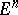, with respect to some fixed systemof points that do not lie in an-dimensional subspace. Every pointcan uniquely be written aswhere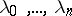are real numbers satisfying the condition. The pointis by definition the centre of gravity of the masseslocated at the points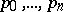. The numbersare called the barycentric coordinates of the point; the point with barycentric coordinates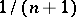is called the barycentre. Barycentric coordinates were introduced by A.F. Möbius in 1827, , as an answer to the question about the masses to be placed at the vertices of a triangle so that a given point is the centre of gravity of these masses. Barycentric coordinates are a special case of homogeneous coordinates; they are affine invariants.

Barycentric coordinates of a simplex are used in algebraic topology . Barycentric coordinates of a point of an-dimensional simplexwith respect to its verticesis the name given to its (ordinary) Cartesian coordinates in the basis of the vectors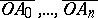, where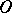is any point that does not lie in the-dimensional subspace carrying(if it is considered thatlies in some Euclidean space, then the definition does not depend on the point), or to projective coordinates with respect to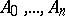in the projective completion of the subspace containing. The barycentric coordinates of the points of a simplex are non-negative and their sum is equal to one. If the-th barycentric coordinate becomes zero, this means that the point lies at the side of the simplexopposite to the vertex. This makes it possible to consider the barycentric coordinates of the points of a geometric complex with respect to all of its vertices. Barycentric coordinates are used to construct the barycentric subdivision of a complex.

Barycentric coordinates of abstract complexes are formally defined in an analogous manner .

How to Cite This Entry:
Barycentric coordinates. E.G. Sklyarenko (originator), Encyclopedia of Mathematics. URL: http://encyclopediaofmath.org/index.php?title=Barycentric_coordinates&oldid=15139
This text originally appeared in Encyclopedia of Mathematics - ISBN 1402006098